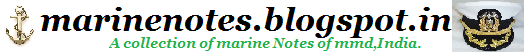Home » » LONGITUDINAL WEIGHT ADDITIONS AND REMOVALS

# LONGITUDINAL WEIGHT ADDITIONS AND REMOVALS

When weight is added or removed to/from a ship, the effects on longitudinal stability are evaluated as well. With the weight change, two things will happen:
1. The ship will sink or rise a few inches
2. The ship will trim about the Center of Flotation (LCF)
The easiest way to calculate the draft changes due to a weight addition/removal not located at LCF is to divide the weight change into two steps. First, assume the weight is added at LCF:The added weight causes the entire ship to sink symmetrically in the water. This is called Parallel Sinkage. If the weight was removed, the ship would rise symmetrically out of the water, called Parallel Rise. To calculate the amount each draft changes due to parallel sinkage/parallel rise (PS/PR) use:Next, transfer the weight from LCF to it's actual location. Although the weight was never really added at LCF then shifted, the end mathematical result will be the same as when the weight was added directly to it�s actual location.This step of moving the weight to it's actual location is identical to a weight shift problem. Again, to determine the change in the ship's drafts due to trim:

1. Calculate the Trimming Moment (TM):2. Calculate the Change in Trim (CT):3. Calculate the change in forward draft (DdFWD):The + or - sign depends on the location of LCF. If LCF is aft of MP use "+" and if LCF is forward of MP use "-".
4. Calculate the change in aft draft (DdAFT):NOTE: If the weight was shifted forward, DdFWD will be positive and DdAFT will be negative. If the weight was shifted aft, DdAFT will be positive and DdFWD will be negative.

The final step is to calculate the total change in draft forward and draft aft by considering both Parallel Rise/Sinkage and change in trim:Example Problem
During VERTREP, all JP-5 in tanks 5-328-0-J and 5-344-0-J is transferred. The Liquid Loading Report shows 57 LT in these tanks prior to transfer. The center of gravity of the combined tanks is 146 FT aft of MP. The CHENG wants to know the new drafts and new trim of the ship. Design Drag is 1'6" by stern.
LBP = 408FT MT1" = 775 FT-Ton/inch TPI = 32.4 LT/inch
DraftFWD = 14'6" DraftAFT = 15'0" LCF = 24FT aft of MP

1. Calculate parallel rise (PR):2. Determine the Trimming Arm (TA):3. Calculate the Trimming Moment (TM):4. Calculate the Change in Trim (CT):5. Calculate the change in draft forward due to trim (DdFWD):6. Calculate the change in draft aft due to trim (DdAFT):Since weight was removed aft, this is a forward trimming moment. The forward draft will increase and the stern draft will decrease.
7. Calculate the total change in draft forward (DDRAFTFWD):8. Calculate the total change in draft aft (DDRAFTAFT):9. Calculate the final drafts and ship's trim:
 FWD Draft AFT Draft Trim Original: 14' 6.00" 15' 0.00" 1'0.00" by the bow Change: +3.25" -5.72" 8.97" by the bow New: 14'9.25" 14' 6.28" 1'8.97" by the bow